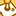# App Development

Announcements
April 22, 2PM EST: Learn about GeoOperations in Qlik Sense SaaS READ MORE
cancel
Showing results for
Did you mean:Partner

## Aggr () as calculated dimension in Bar chart

Hello,

I'm having trouble with creating a calculated dimension for a histogram in Qliksense.

As shown in the image below,  I was hoping to create a set of ranges on the x-axis : 0%, 1~5%, 6~10%, 11~15%, etc. However, for some reason, the chart will not generate 1~5%  range and instead, those data points are grouped with 0%.  For instance, the value of 0.0188 will be grouped into 0% (as shown in the image below in Aggr Expr - Dimension column). Funny thing is that when I put the same expression as a measure (Aggr Expr - Measure), it seems to be working fine.
What could be happening here?This is the expression I used to calculate those ranges:

=aggr(
if(
only({\$} TEST_PERCENTAGE) = 0, dual('0%', 0)
, dual(
num((div(only({\$} TEST_PERCENTAGE) - 0.01, 0.05) * 5) + 1, '###0') & '-' & num((div(only({\$}                                           TEST_PERCENTAGE) -  0.01, 0.05) + 1) * 0.05, '###0%')
,  div(only({\$} TEST_PERCENTAGE) - 0.01, 0.05
)
)
), TEST_PERCENTAGE
)

Labels (3)

• ### Calculated dimension

0 Replies